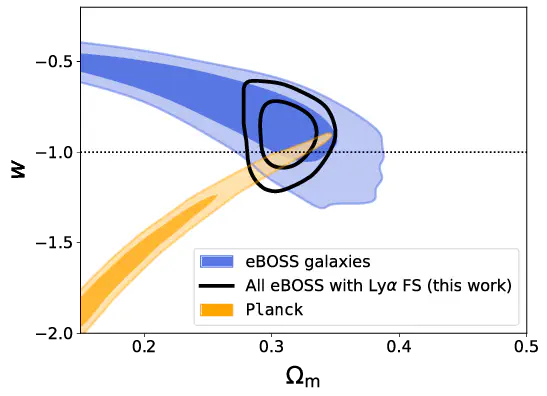# New constraints on the expansion rate at redshift 2.3 from the Lyman-$α$ forest### Abstract

We measure the expansion rate of the Universe at redshift z=2.3 from the anisotropy of Lyman-α (Lyα) forest correlations measured by the Sloan Digital Sky Survey (SDSS). Our result is the most precise from large-scale structure at z>1. In flat ΛCDM we determine the matter density to be Ωm=0.36+0.03−0.04 from Lyα alone, a factor of two tighter than baryon acoustic oscillation results from the same data. Using a nucleosynthesis prior, we measure the Hubble constant to be H0=63.2±2.5 km/s/Mpc. In combination with other SDSS tracers, we find H0=67.2±0.9 km/s/Mpc and measure the dark energy equation-of-state parameter to be w=−0.90±0.12. Our work opens a new avenue for constraining cosmology at high redshift.

Publication
arXiv